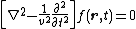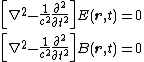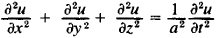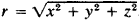# Wave Equation

Also found in: Dictionary, Thesaurus, Wikipedia.

## Wave equation

The name given to certain partial differential equations in classical and quantum physics which relate the spatial and time dependence of physical functions. In this article the classical and quantum wave equations are discussed separately, with the classical equations first for historical reasons.

In classical physics the name wave equation is given to the linear, homogeneous partial differential equations which have the form of Eq. (1).

(1)Here &ugr; is a parameter with the dimensions of velocity; r represents the space coordinates x, y, z; t is the time; and ∇2 is Laplace's operator defined by
(2)Eq. (2). The function f( r ,t) is a physical observable; that is, it can be measured and consequently must be a real function.

The simplest example of a wave equation in classical physics is that governing the transverse motion of a string under tension and constrained to move in a plane.

A second type of classical physical situation in which the wave equation (1) supplies a mathematical description of the physical reality is the propagation of pressure waves in a fluid medium. Such waves are called acoustical waves, the propagation of sound being an example. A third example of a classical physical situation in which Eq. (1) gives a description of the phenomena is afforded by electromagnetic waves. In a region of space in which the charge and current densities are zero, Maxwell's equations for the photon lead to the wave equations (3).

(3)Here E is the electric field strength and B is the magnetic flux density; they are both vectors in ordinary space. The parameter c is the speed of light in vacuum. See Electromagnetic radiation, Maxwell's equations

The nonrelativistic Schrödinger equation is an example of a quantum wave equation. Relativistic quantum-mechanical wave equations include the Schrödinger-Klein-Gordon equation and the Dirac equation. See Quantum mechanics, Relativistic quantum theory

## Wave Equation

a partial differential equation that describes the process of propagation of a disturbance in a medium. In the case of small disturbances and a homogeneous, isotropic medium, the wave equation has the formwhere x, y, and z are spatial variables; t is time; u = u(x, y, z) is the function to be determined, which characterizes the disturbance at point (x, y, z) and time t; and a is the velocity of propagation of the disturbance. The wave equation is one of the fundamental equations of mathematical physics and is applied extensively. If u is a function of only two (one) spatial variables, then the wave equation is simplified and is called a two-dimensional (one-dimensional) equation. It permits a solution in the form of a“diverging spherical wave”:

u = f(t – r/a)/r

where f is an arbitrary function and. The so-called elementary solution (elementary wave) is of particular interest:

u = δ(t - r/a)/r

(where δ is the delta function); it gives the process of propagation for a disturbance produced by an instantaneous point source acting at the origin (when t = 0). Figuratively speaking, an elementary wave is an“infinite surge” on a circumference r = at that is moving away from the origin at a velocity a with gradually diminishing intensity. By superimposing elementary waves it is possible to describe the process of propagation of an arbitrary disturbance.

Small vibrations of a string are described by a one-dimensional wave equation:In 1747, J. d’Alembert proposed a method of solving this wave equation in terms of superimposed forward and back waves: u = f(x - at) + g(x + at); and in 1748, L. Euler established that the functions f and g are determined by as-signing so-called initial conditions.

### REFERENCE

Tikhonov, A. N., and A. A. Samarskii. Uravneniia matematicheskoi fiziki, 3rd ed. Moscow, 1966.

P. I. LIZORKIN

## wave equation

[′wāv i‚kwā·zhən]
(physics)
In classical physics, a special equation governing waves that suffer no dissipative attenuation; it states that the second partial derivative with respect to time of the function characterizing the wave is equal to the square of the wave velocity times the Laplacian of this function. Also known as classical wave equation; d'Alembert's wave equation.
Any of several equations which relate the spatial and time dependence of a function characterizing some physical entity which can propagate as a wave, including quantum-wave equations for particles.
References in periodicals archive ?
Numerical solution of regularized long wave equation by reduced differential transform method.
Zhao, "Jacobi elliptic function expansion method and periodic wave solutions of nonlinear wave equations," Physics Letters A, vol.
At last, returning to (16), using double integration operation in (16), the general solution of the transformed wave equation (10) for the third version is
In consequence, the wave motion equation of the bar with material fields can also be equivalent to the cylindrical wave equation.
In a nutshell, the wave equation (1) describes the propagation of an excitation generated by initial or boundary condition with a constant speed [c.
0], the excitation frequency f = 1GHz, and I = 1mA, the exact solution may be obtained by solving the wave equation in the spectral domain as presented in Appendix A.
X,Y,Z] to meet wave equation similar to (4), but it is needless to do because in the next section a mathematical proof will be done.
s,[theta]] space [6,7,11] associated to the wave equation and the nonlinear term estimates in [X.
1994) (42) in deriving a strongly nonlinear, strongly dispersive wave equation by applying the Galerkin method to the Euler equations of motion.
Whereas leptons are the fundamental and excited eigenvectors of the Wave Equation as shown in the Appendix in Ref.
Sumit Gupta, senior director of the Tesla business at NVIDIA, said: "The 28X acceleration Spectraseis is seeing in elastic wave equation imaging underscores the tremendous benefits GPUs provide to the oil and gas industry.
The measurements - 10 times more accurate than any previously reported - at Sandia's Z accelerator agree with results from a modern simulation effort that uses the quantum mechanics of Schrodinger's wave equation - the fundamental equation of wave mechanics - to predict the behaviour of water under extreme pressure and density.

Site: Follow: Share:
Open / Close